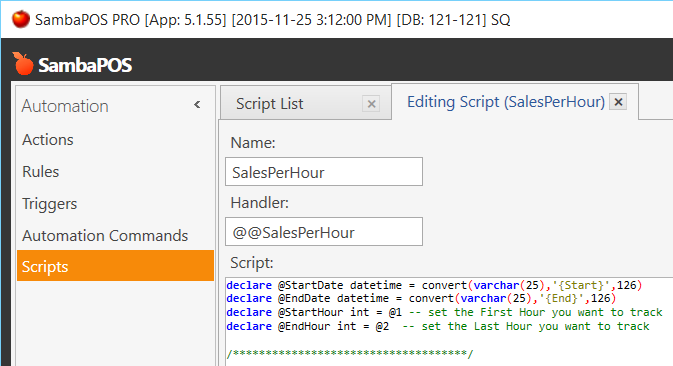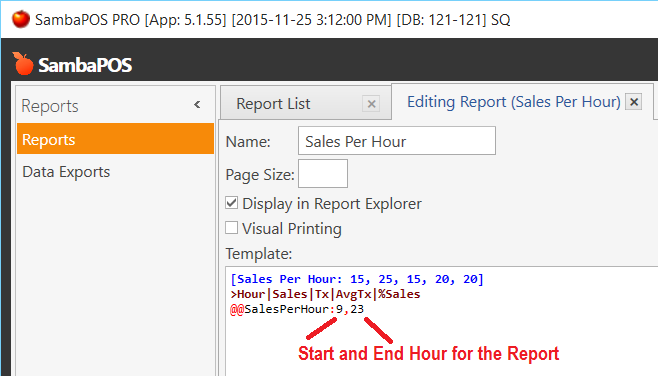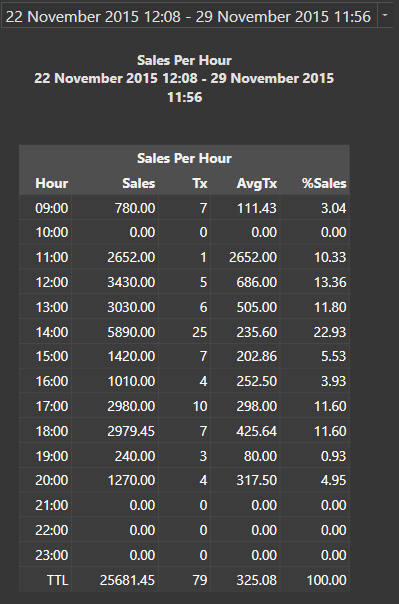# Exporting certain values to text (reports?)

Hi,

I am wondering if there is an easy way to:

1. Create an hour by hour sales report
2. Export the total sales for the day and month to a text file, for example, just the total sales value to text or email.

Is there any way to do this?

Thank you,

You need to be much more specific as to your exact needs. To answer your question: yes. You can create nearly any report you desire using Custom Reports and/or SQL, and yes, you can save and email those reports.`@@SalesPerHour`

``````declare @StartDate datetime = convert(varchar(25),'{Start}',126)
declare @EndDate datetime = convert(varchar(25),'{End}',126)
declare @StartHour int = @1 -- set the First Hour you want to track
declare @EndHour int = @2  -- set the Last Hour you want to track

/************************************/

declare @Hour int = 0
declare @TotalSales money = 0.00

declare @tbl_Sales table
(
[ID]  INT IDENTITY(1,1) NOT NULL
,[Hour] varchar(5)
,[Amount] decimal(9,2)
,[Tickets] int
,[AvgTx] decimal(9,2)
,[Percent] decimal(9,2)
)

set @Hour = @StartHour

WHILE @Hour < @EndHour+1
BEGIN
INSERT INTO @tbl_Sales
SELECT
@Hour as [Hour]
, SUM(CASE WHEN (DATEPART(hour,[Date])>=@Hour AND DATEPART(hour,[Date])<@Hour+1)
THEN [TotalAmount]
ELSE 0 END) as [Amount]
, COUNT(CASE WHEN (DATEPART(hour,[Date])>=@Hour AND DATEPART(hour,[Date])<@Hour+1)
THEN 1
ELSE null END) as [Tickets]
,0,0
FROM [dbo].[Tickets]
WHERE [Date]>=@StartDate AND [Date]<=@EndDate
SET @Hour = @Hour + 1
END

INSERT INTO @tbl_Sales
SELECT 'TTL', sum([Amount]), sum([Tickets]), 0, 0 FROM @tbl_Sales

set @TotalSales = (SELECT [Amount] FROM @tbl_Sales WHERE [Hour]='TTL')

UPDATE @tbl_Sales SET
[AvgTx] = (CASE WHEN [Tickets]=0 THEN 0 ELSE [Amount]/[Tickets] END)
,[Percent] = [Amount]/@TotalSales * 100

SELECT CASE
WHEN isnumeric([Hour])=1
THEN (CASE WHEN [Hour]<10 THEN '0'+[Hour]+':00' ELSE [Hour]+':00' END)
ELSE [Hour]
END as [Hour]
, [Amount], [Tickets], [AvgTx], [Percent]
FROM @tbl_Sales
```````Sales Per Hour`

``````[Sales Per Hour: 15, 25, 15, 20, 20]
>Hour|Sales|Tx|AvgTx|%Sales
@@SalesPerHour:9,23
``````

###OutputYou can also use the Data Exports feature to have Report Data written to other file types such as CSV and XML.

2 Likes

This is exactly what I needed. Works wonders, thanks x 1000!